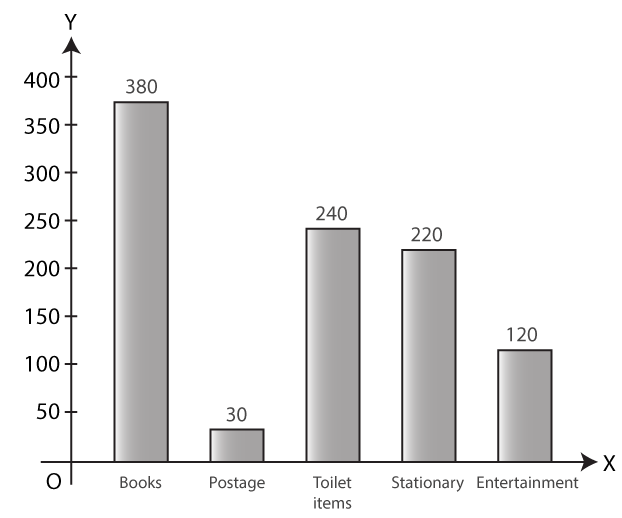# Selina Solutions Concise Mathematics Class 6 Chapter 33 Data Handling Exercise 33(C)

Selina Solutions Concise Mathematics Class 6 Chapter 33 Data Handling Exercise 33(C) contains accurate answers to problems for each and every question in this exercise. Representing the given data in a bar graph is the major concept covered here. Students who practice the solutions, prepared by experts, enhance problem solving abilities within a short duration. This also helps them to perform better in academics as well. To obtain more conceptual knowledge, students can refer to solutions both online and offline, whenever required. In order to clear their confusion, students can make use of Selina Solutions Concise Mathematics Class 6 Chapter 33 Data Handling Exercise 33(C) PDF, from the links presented here, with a free download option.

## Selina Solutions Concise Mathematics Class 6 Chapter 33 Data Handling Exercise 33(C) Download PDF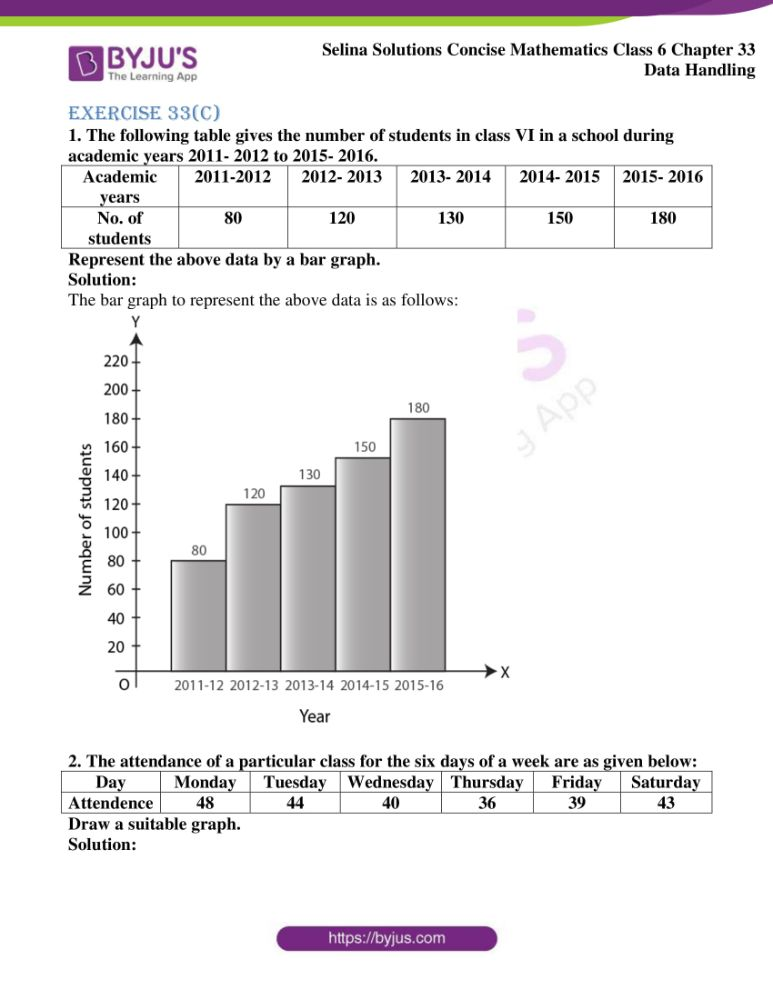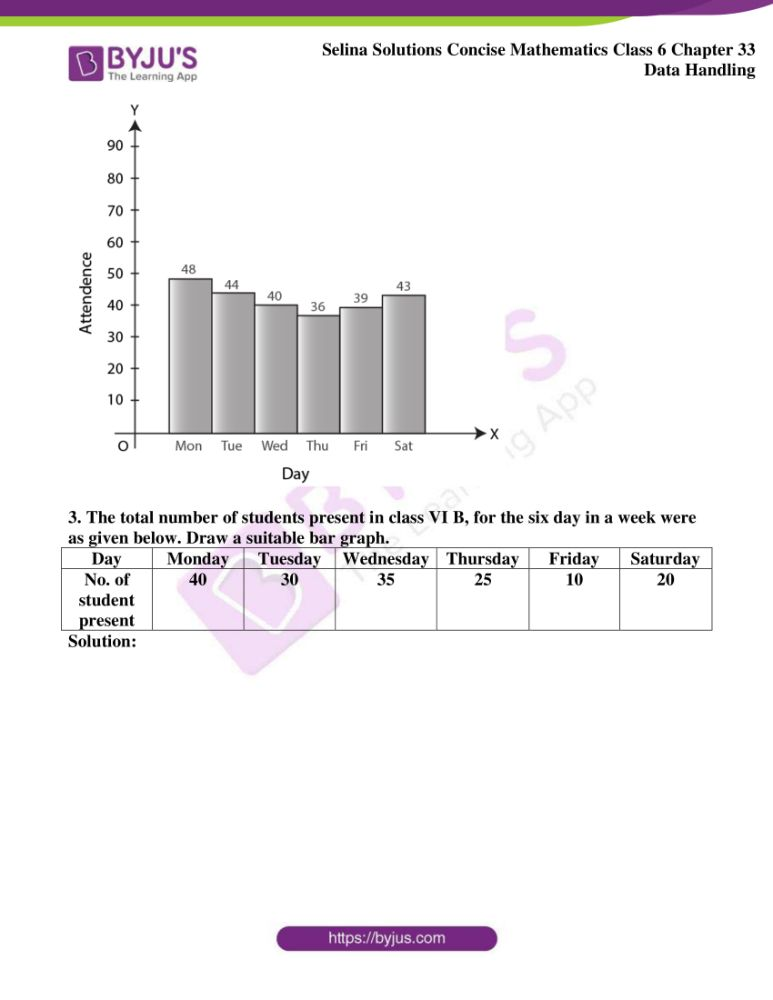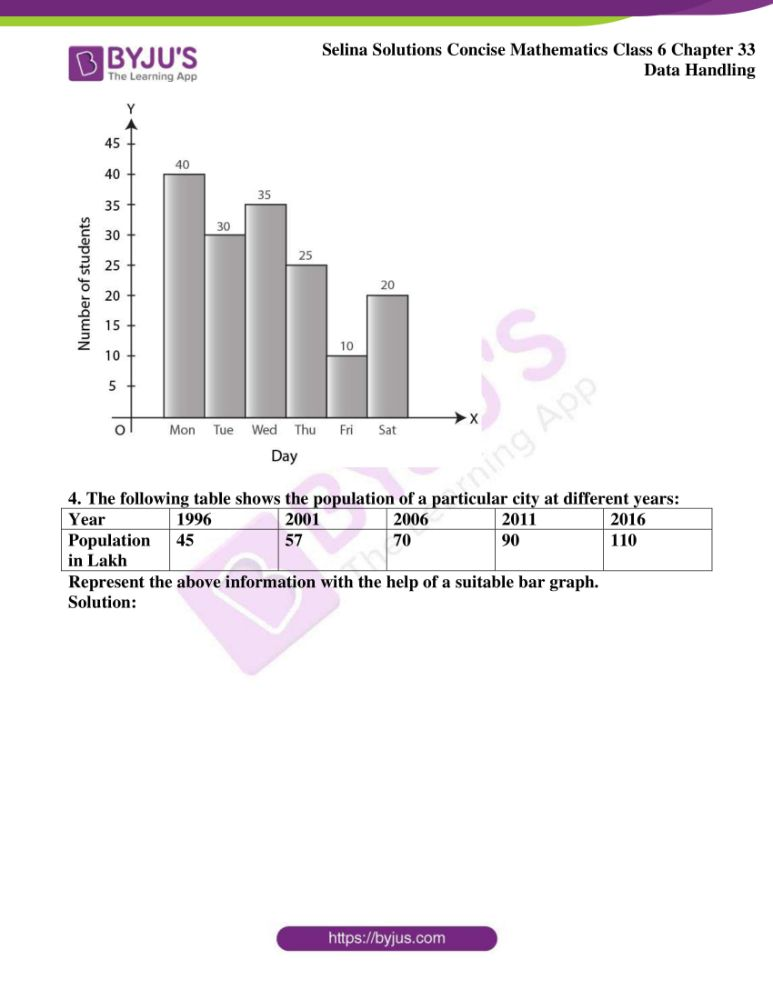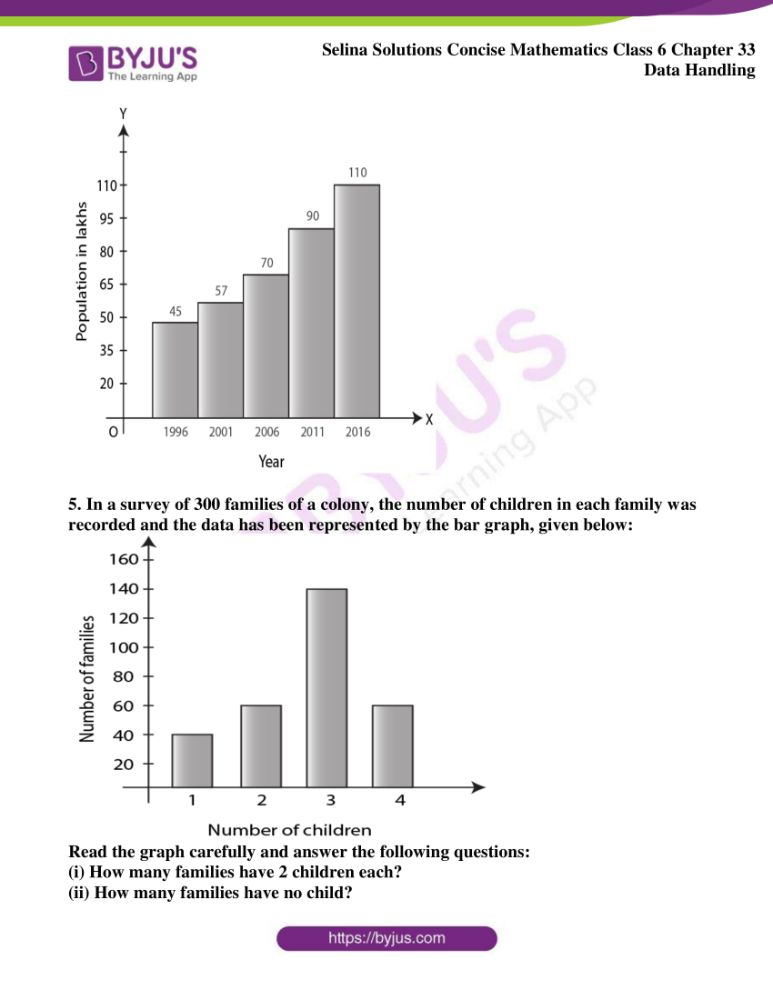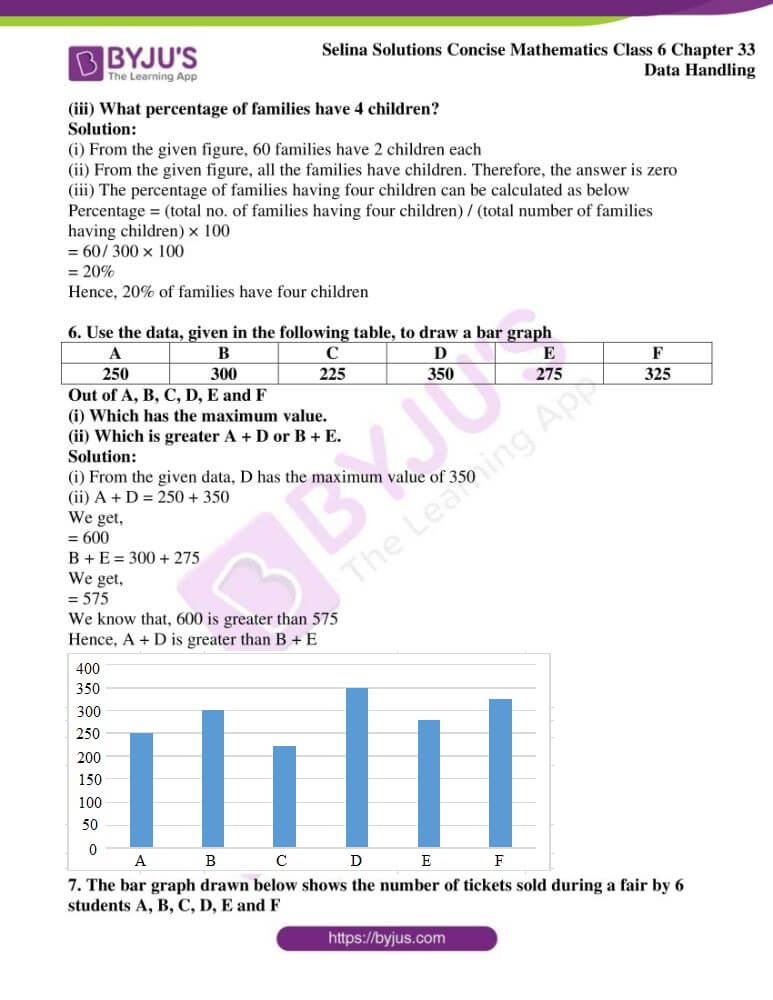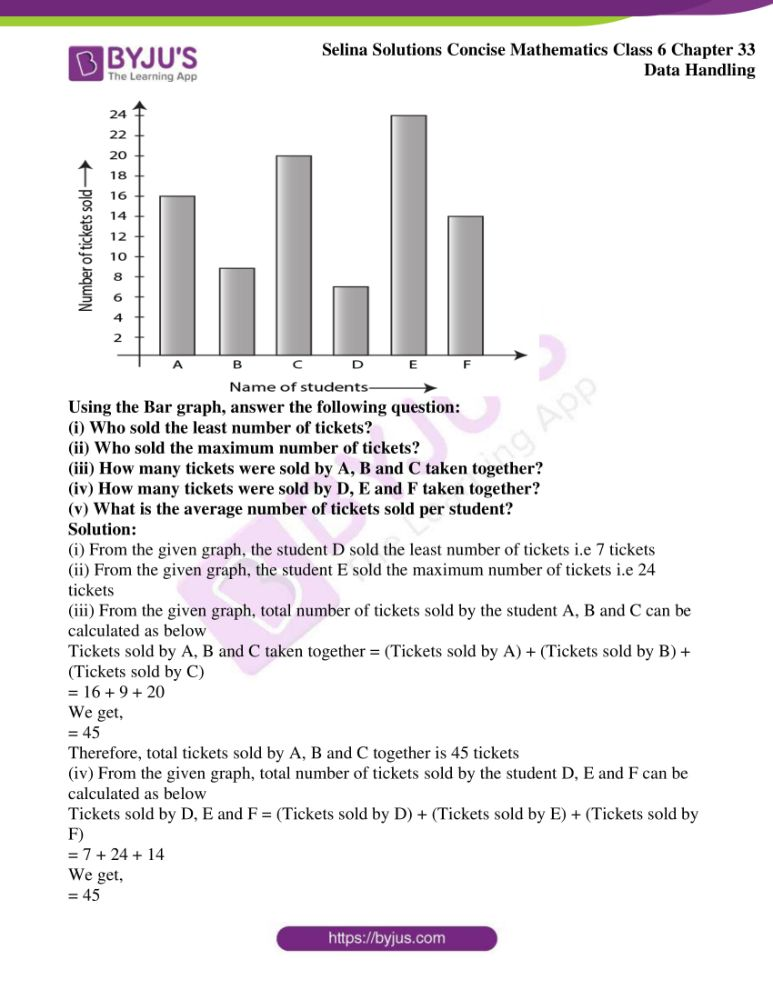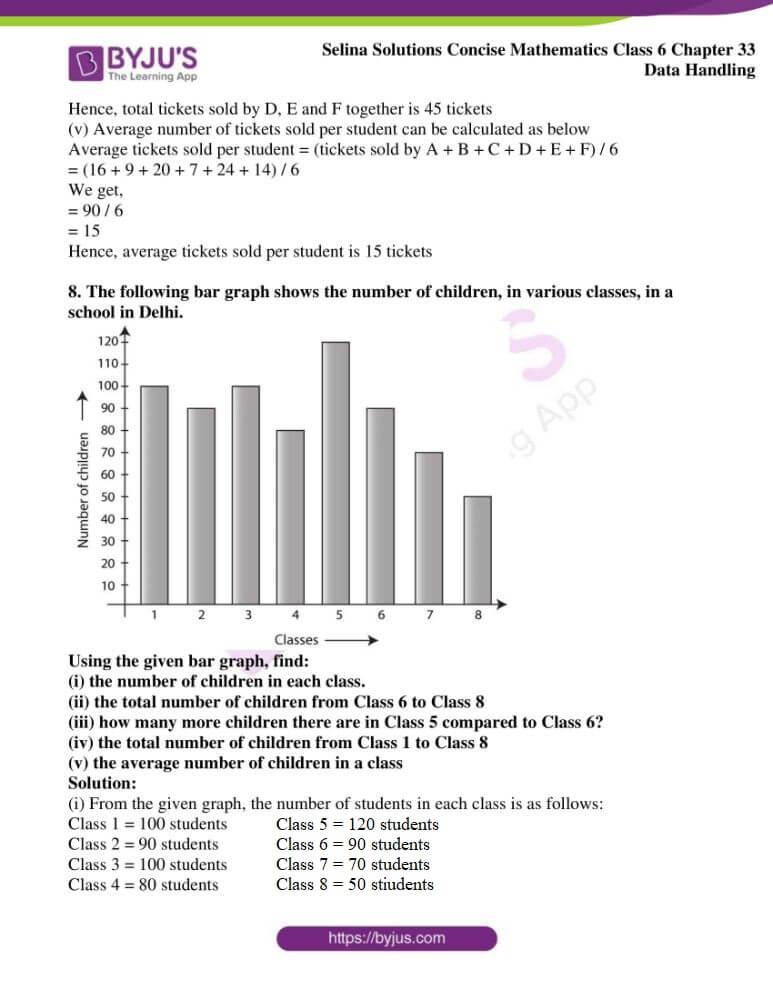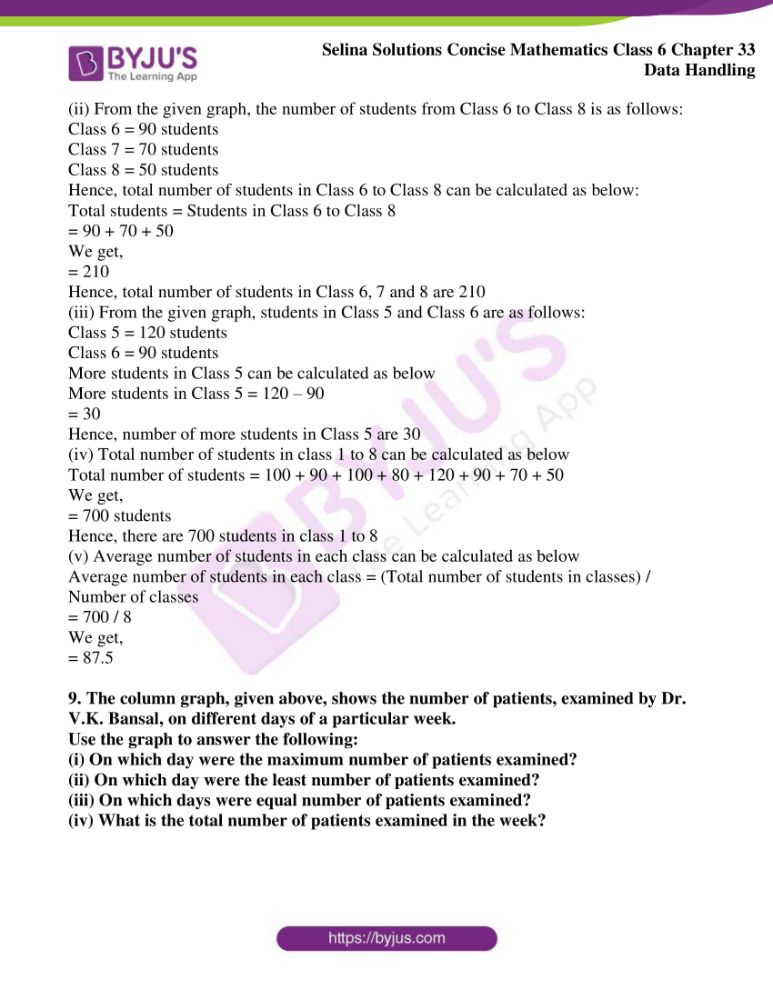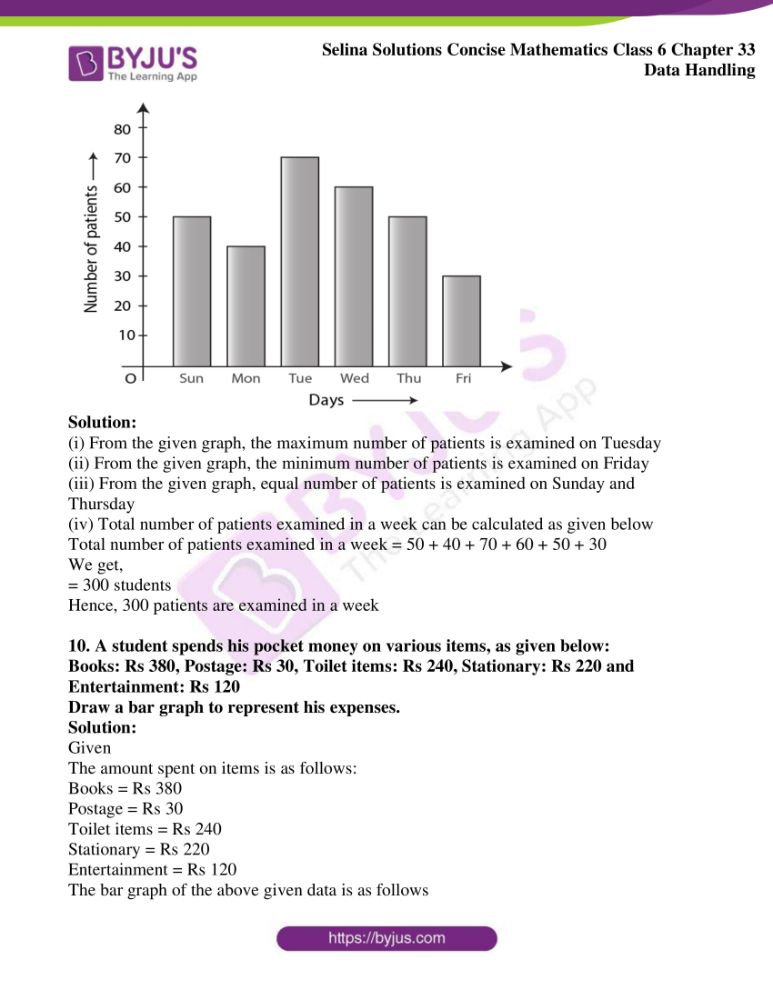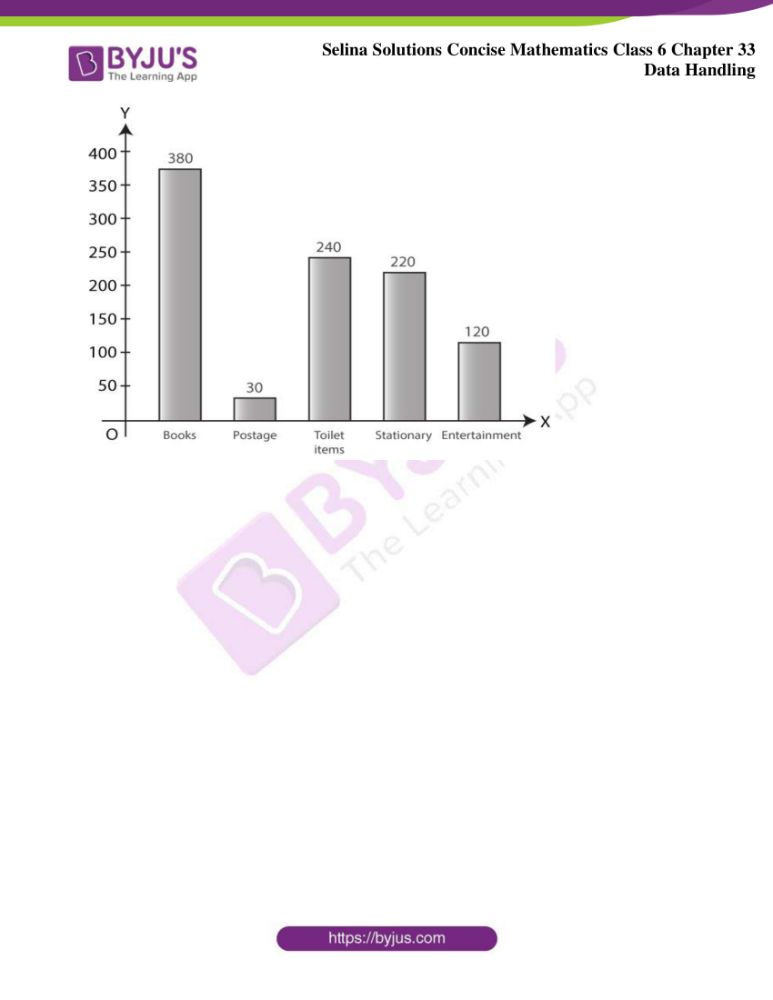### Access other exercises of Selina Solutions Concise Mathematics Class 6 Chapter 33 Data Handling

Exercise 33(A) Solutions

Exercise 33(B) Solutions

### Access Selina Solutions Concise Mathematics Class 6 Chapter 33 Data Handling Exercise 33(C)

Exercise 33(C)

1. The following table gives the number of students in class VI in a school during academic years 2011- 2012 to 2015- 2016.

 Academic years 2011-2012 2012- 2013 2013- 2014 2014- 2015 2015- 2016 No. of students 80 120 130 150 180

Represent the above data by a bar graph.

Solution:

The bar graph to represent the above data is as follows: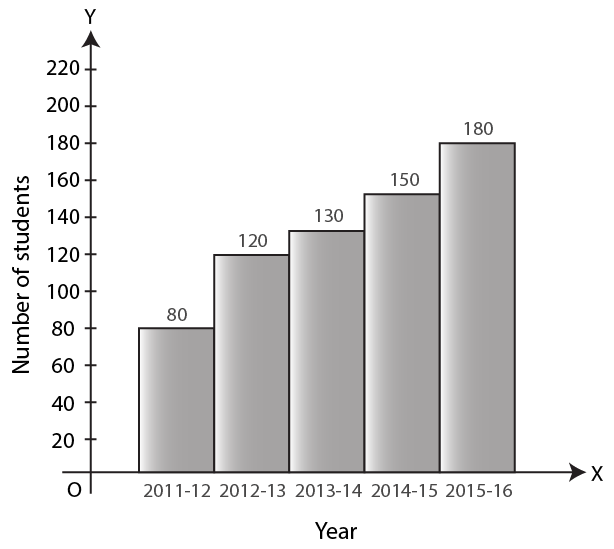2. The attendance of a particular class for the six days of a week are as given below:

 Day Monday Tuesday Wednesday Thursday Friday Saturday Attendence 48 44 40 36 39 43

Draw a suitable graph.

Solution: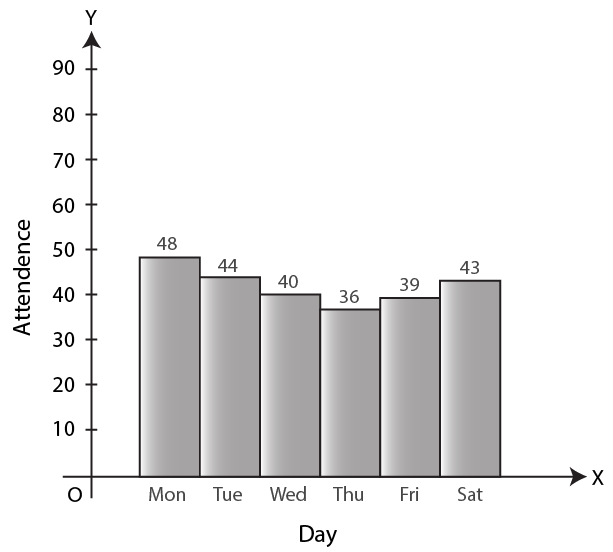3. The total number of students present in class VI B, for the six day in a week were as given below. Draw a suitable bar graph.

 Day Monday Tuesday Wednesday Thursday Friday Saturday No. of student present 40 30 35 25 10 20

Solution: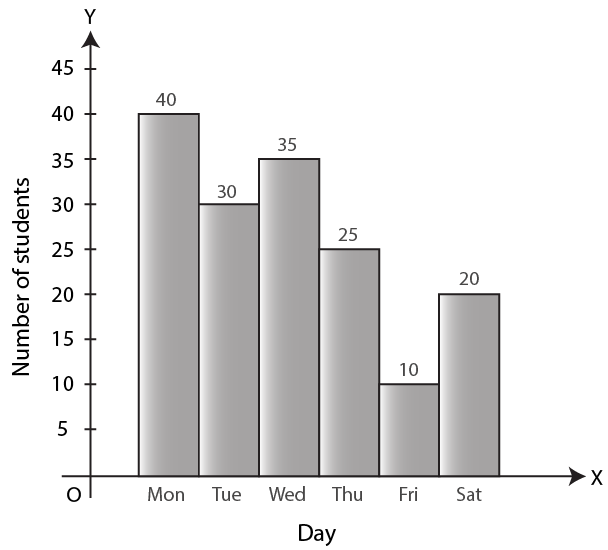4. The following table shows the population of a particular city at different years:

 Year 1996 2001 2006 2011 2016 Population in Lakh 45 57 70 90 110

Represent the above information with the help of a suitable bar graph.

Solution: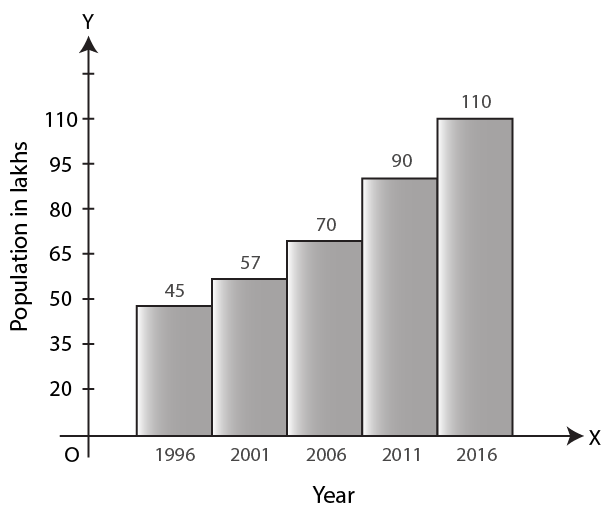5. In a survey of 300 families of a colony, the number of children in each family was recorded and the data has been represented by the bar graph, given below: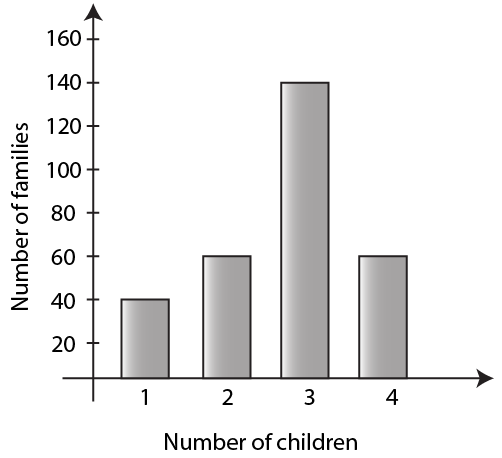(i) How many families have 2 children each?

(ii) How many families have no child?

(iii) What percentage of families have 4 children?

Solution:

(i) From the given figure, 60 families have 2 children each

(ii) From the given figure, all the families have children. Therefore, the answer is zero

(iii) The percentage of families having four children can be calculated as below

Percentage = (total no. of families having four children) / (total number of families having children) × 100

= 60 / 300 × 100

= 20%

Hence, 20% of families have four children

6. Use the data, given in the following table, to draw a bar graph

 A B C D E F 250 300 225 350 275 325

Out of A, B, C, D, E and F

(i) Which has the maximum value.

(ii) Which is greater A + D or B + E.

Solution: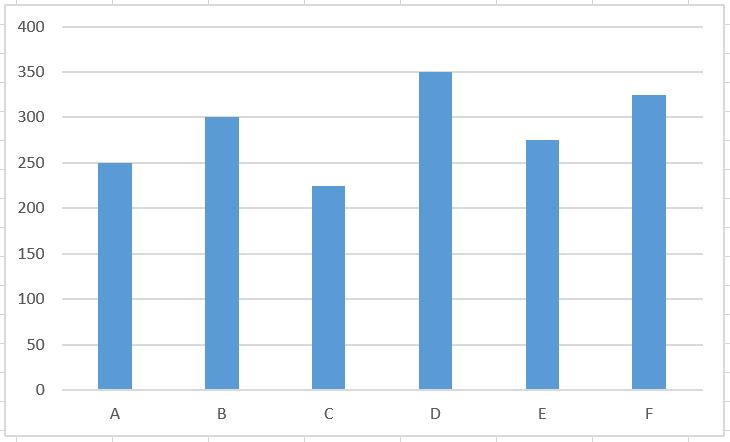(i) From the given data, D has the maximum value of 350

(ii) A + D = 250 + 350

We get,

= 600

B + E = 300 + 275

We get,

= 575

We know that, 600 is greater than 575

Hence, A + D is greater than B + E

7. The bar graph drawn below shows the number of tickets sold during a fair by 6 students A, B, C, D, E and F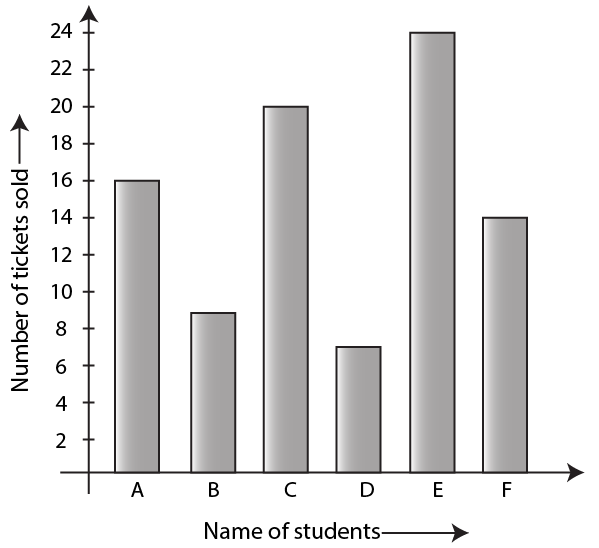Using the Bar graph, answer the following question:

(i) Who sold the least number of tickets?

(ii) Who sold the maximum number of tickets?

(iii) How many tickets were sold by A, B and C taken together?

(iv) How many tickets were sold by D, E and F taken together?

(v) What is the average number of tickets sold per student?

Solution:

(i) From the given graph, the student D sold the least number of tickets i.e 7 tickets

(ii) From the given graph, the student E sold the maximum number of tickets i.e 24 tickets

(iii) From the given graph, total number of tickets sold by the student A, B and C can be calculated as below

Tickets sold by A, B and C taken together = (Tickets sold by A) + (Tickets sold by B) + (Tickets sold by C)

= 16 + 9 + 20

We get,

= 45

Therefore, total tickets sold by A, B and C together is 45 tickets

(iv) From the given graph, total number of tickets sold by the student D, E and F can be calculated as below

Tickets sold by D, E and F = (Tickets sold by D) + (Tickets sold by E) + (Tickets sold by F)

= 7 + 24 + 14

We get,

= 45

Hence, total tickets sold by D, E and F together is 45 tickets

(v) Average number of tickets sold per student can be calculated as below

Average tickets sold per student = (tickets sold by A + B + C + D + E + F) / 6

= (16 + 9 + 20 + 7 + 24 + 14) / 6

We get,

= 90 / 6

= 15

Hence, average tickets sold per student is 15 tickets

8. The following bar graph shows the number of children, in various classes, in a school in Delhi.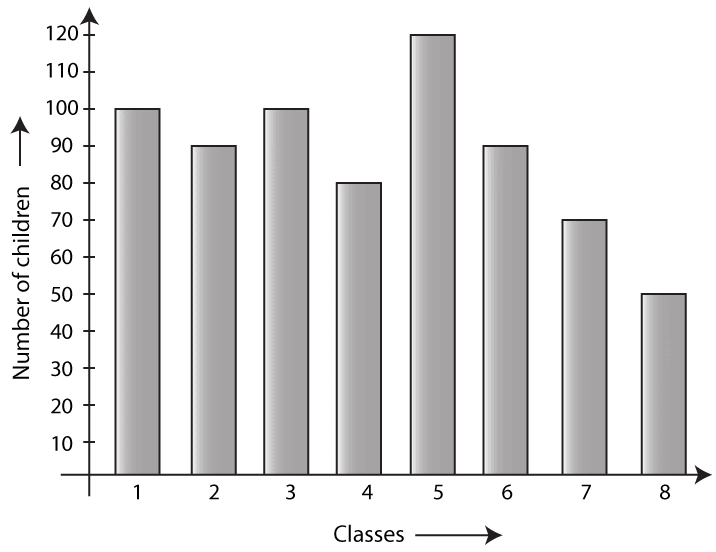Using the given bar graph, find:

(i) the number of children in each class.

(ii) the total number of children from Class 6 to Class 8

(iii) how many more children there are in Class 5 compared to Class 6?

(iv) the total number of children from Class 1 to Class 8

(v) the average number of children in a class

Solution:

(i) From the given graph, the number of students in each class is as follows:

Class 1 = 100 students

Class 2 = 90 students

Class 3 = 100 students

Class 4 = 80 students

Class 5 = 120 students

Class 6 = 90 students

Class 7 = 70 students

Class 8 = 50 students

(ii) From the given graph, the number of students from Class 6 to Class 8 is as follows:

Class 6 = 90 students

Class 7 = 70 students

Class 8 = 50 students

Hence, total number of students in Class 6 to Class 8 can be calculated as below:

Total students = Students in Class 6 to Class 8

= 90 + 70 + 50

We get,

= 210

Hence, total number of students in Class 6, 7 and 8 are 210

(iii) From the given graph, students in Class 5 and Class 6 are as follows:

Class 5 = 120 students

Class 6 = 90 students

More students in Class 5 can be calculated as below

More students in Class 5 = 120 – 90

= 30

Hence, number of more students in Class 5 are 30

(iv) Total number of students in class 1 to 8 can be calculated as below

Total number of students = 100 + 90 + 100 + 80 + 120 + 90 + 70 + 50

We get,

= 700 students

Hence, there are 700 students in class 1 to 8

(v) Average number of students in each class can be calculated as below

Average number of students in each class = (Total number of students in classes) / Number of classes

= 700 / 8

We get,

= 87.5

9. The column graph, given above, shows the number of patients, examined by Dr. V.K. Bansal, on different days of a particular week.

Use the graph to answer the following:

(i) On which day were the maximum number of patients examined?

(ii) On which day were the least number of patients examined?

(iii) On which days were equal number of patients examined?

(iv) What is the total number of patients examined in the week?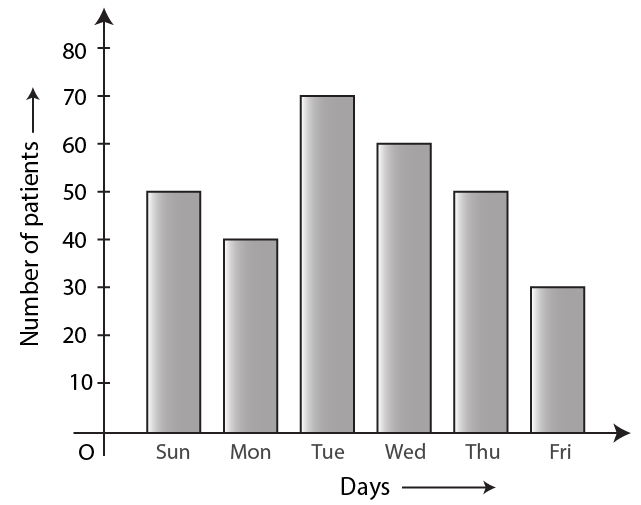Solution:

(i) From the given graph, the maximum number of patients is examined on Tuesday

(ii) From the given graph, the minimum number of patients is examined on Friday

(iii) From the given graph, equal number of patients is examined on Sunday and Thursday

(iv) Total number of patients examined in a week can be calculated as given below

Total number of patients examined in a week = 50 + 40 + 70 + 60 + 50 + 30

We get,

= 300 students

Hence, 300 patients are examined in a week

10. A student spends his pocket money on various items, as given below:

Books: Rs 380, Postage: Rs 30, Toilet items: Rs 240, Stationary: Rs 220 and Entertainment: Rs 120

Draw a bar graph to represent his expenses.

Solution:

Given

The amount spent on items is as follows:

Books = Rs 380

Postage = Rs 30

Toilet items = Rs 240

Stationary = Rs 220

Entertainment = Rs 120

The bar graph of the above given data is as follows